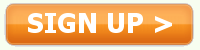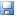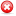# Fractal Geometry

" is not just a chapter of mathematics, but one that helps
Everyman to see the same old world differently". - Benoit Mandelbrot
The world of mathematics usually tends to be thought of as abstract. Complex and
imaginary numbers, real numbers, logarithms, functions, some tangible and others
imperceivable. But these abstract numbers, simply symbols that conjure an image,
a quantity, in our mind, and complex equations, take on a new meaning with
fractals - a concrete one. Fractals go from being very simple equations on a
piece of paper to colorful, extraordinary images, and most of all, offer an
explanation to things. The importance of fractal geometry is that it provides an

 Want to read the rest of this paper?Join Essayworld today to view this entire essayand over 50,000 other term papers

palette of subjects in life, and
science - the physical, the abstract, and the natural.

We were all astounded by the sudden revelation that the output of a
very simple, two-line generating formula does not have to be a dry and
cold abstraction. When the output was what is now called a fractal,
no one called it artificial... Fractals suddenly broadened the realm
in which understanding can be based on a plain physical basis.
(McGuire, Foreword by Benoit Mandelbrot)

A fractal is a geometric shape that is complex and detailed at every level of
magnification, as well as self-similar. Self-similarity is something looking the
same over all ranges of scale, meaning a small portion of a fractal can be
viewed as a microcosm of the larger fractal. One of the simplest examples of a
fractal is the snowflake. It is constructed by taking an equilateral triangle,
and after many iterations of adding smaller triangles to ...

 Get instant access to over 50,000 essays.Write better papers. Get better grades.Already a member? Login

rougher, the more filling of that space it is.
(McGuire, p. 14)

Fractal Dimensions are an attempt to measure, or define the pattern, in fractals.
A zero-dimensional universe is one point. A one-dimensional universe is a single
line, extending infinitely. A two-dimensional universe is a plane, a flat
surface extending in all directions, and a three-dimensional universe, such as
ours, extends in all directions. All of these dimensions are defined by a whole
number. What, then, would a 2.5 or 3.2 dimensional universe look like? This is
answered by fractal geometry, the word fractal coming from the concept of
fractional dimensions. ...

 Succeed in your coursework without stepping into a library.Get access to a growing library of notes, book reports,and research papers in 2 minutes or less.

Fractal Geometry. (2007, January 27). Retrieved October 22, 2021, from http://www.essayworld.com/essays/Fractal-Geometry/59376
"Fractal Geometry." Essayworld.com. Essayworld.com, 27 Jan. 2007. Web. 22 Oct. 2021. <http://www.essayworld.com/essays/Fractal-Geometry/59376>
"Fractal Geometry." Essayworld.com. January 27, 2007. Accessed October 22, 2021. http://www.essayworld.com/essays/Fractal-Geometry/59376.
"Fractal Geometry." Essayworld.com. January 27, 2007. Accessed October 22, 2021. http://www.essayworld.com/essays/Fractal-Geometry/59376.
 PAPER DETAILS Added: 1/27/2007 04:33:15 PMCategory: Education Type: Premium PaperWords: 1456Pages: 6Save |Report